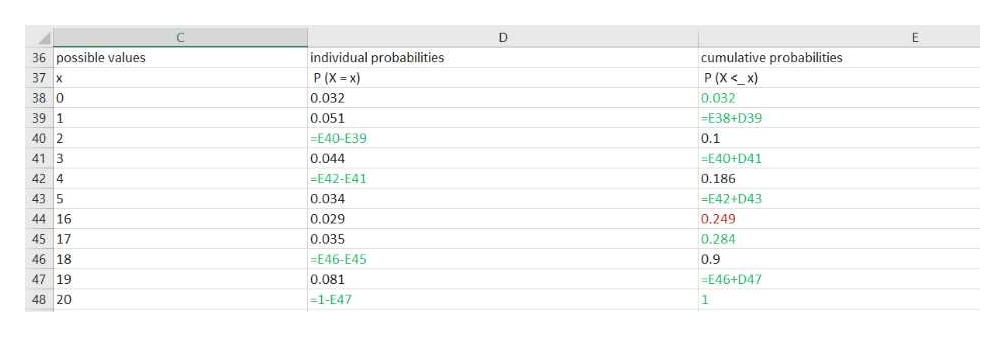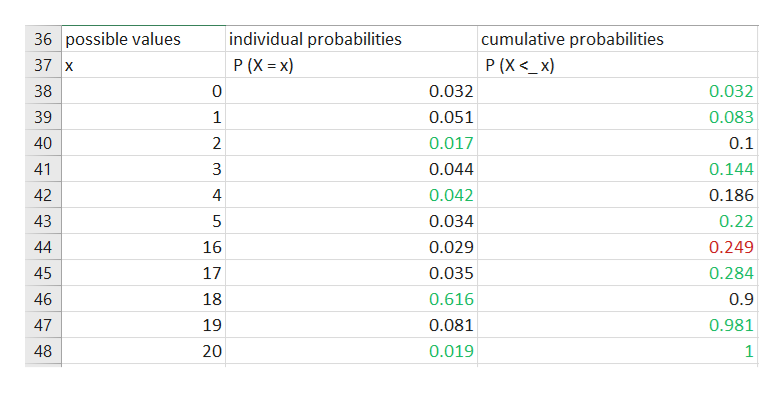A table is shown below that shows some of the individual and/or cumulative probabilities for a discrete random variable which has a possible values at all integers from 0-20.a) fill in the missing values that you can find for the table from the given information. FInd the probability that X is ...b) ...at most 18c) ...less than d) ...less thanf) ...more than 4g) ...between 4 and 16, inclusivepossible valuesindividual probabilitiescumulative probabilitiesx P (X = x) P (X <_ x)0.032 1.051 2 .1003.044 4 .1865.034 16.029..81617.035 18 .90019.081 20

Question

A table is shown below that shows some of the individual and/or cumulative probabilities for a discrete random variable which has a possible values at all integers from 0-20.

a) fill in the missing values that you can find for the table from the given information.

FInd the probability that X is ...

b) ...at most 18
c) ...less than

d) ...less than

f) ...more than 4

g) ...between 4 and 16, inclusive

 possible values individual probabilities cumulative probabilities x P (X = x) P (X <_ x) 0 .032 1 .051 2 .100 3 .044 4 .186 5 .034 16 .029. .816 17 .035 18 .900 19 .081 20
Step 1

Enter the data in the excel same as given in the question  and the following formulas in the blanks to get the resultshelp_outlineImage TranscriptioncloseC E individual probabilities 36 possible values cumulative probabilities P (X
Step 2

In discrete random variable

Step 3

The following result is obtained

The missing values is shown in green colour,...help_outlineImage Transcriptionclose36 possible values individual probabilities cumulative probabilities 37 x P (X<_x) P (X x) 0.032 38 0 0.032 39 1 0.051 0.083 40 2 0.017 0.1 41 3 0.044 0.144 0.186 42 4 0.042 43 5 0.034 0.22 44 16 0.029 0.249 0.284 45 17 0.035 46 18 0.616 0.9 47 19 0.081 0.981 0.019 48 20 1 st LC LC fullscreen

Want to see the full answer?

See Solution

Want to see this answer and more?

Our solutions are written by experts, many with advanced degrees, and available 24/7

See Solution
Tagged in

Measures